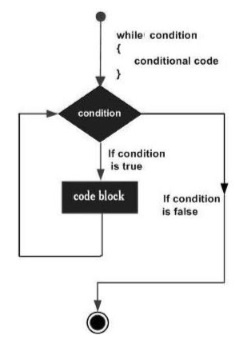# Groovy - While Statement

The syntax of the while statement is shown below −

```while(condition) {
statement #1
statement #2
...
}
```

The while statement is executed by first evaluating the condition expression (a Boolean value), and if the result is true, then the statements in the while loop are executed. The process is repeated starting from the evaluation of the condition in the while statement. This loop continues until the condition evaluates to false. When the condition becomes false, the loop terminates. The program logic then continues with the statement immediately following the while statement. The following diagram shows the diagrammatic explanation of this loop.Following is an example of a while loop statement −

```class Example {
static void main(String[] args) {
int count = 0;

while(count<5) {
println(count);
count++;
}
}
}
```

In the above example, we are first initializing the value of a count integer variable to 0. Then our condition in the while loop is that we are evaluating the condition of the expression to be that count should be less than 5. Till the value of count is less than 5, we will print the value of count and then increment the value of count. The output of the above code would be −

```0
1
2
3
4
```
groovy_loops.htm